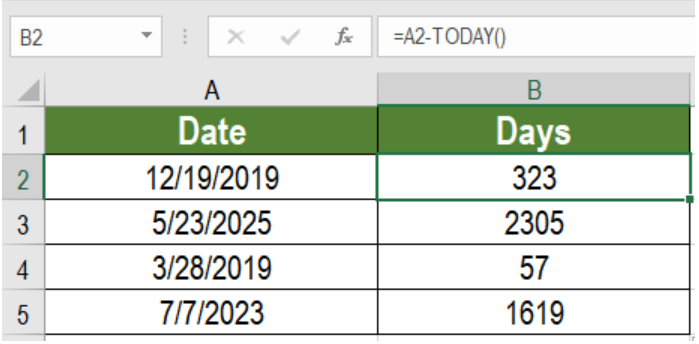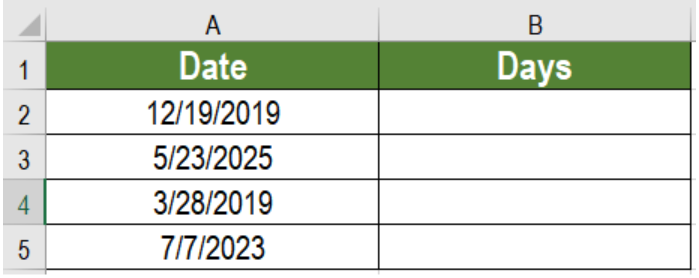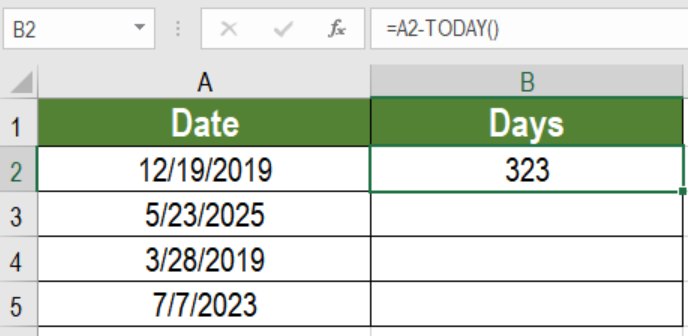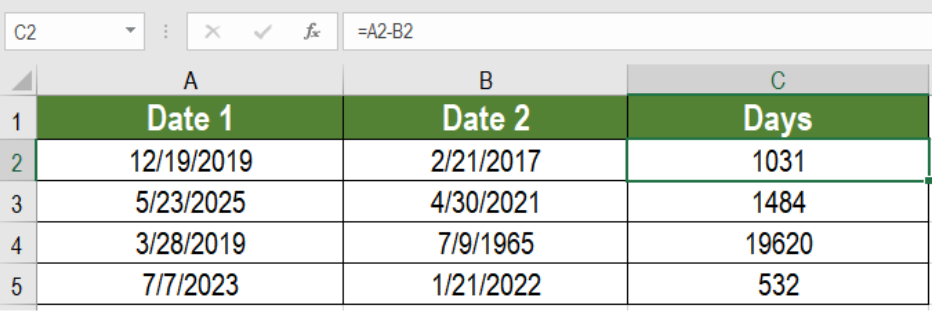Get instant live expert help with Excel or Google Sheets“My Excelchat expert helped me in less than 20 minutes, saving me what would have been 5 hours of work!”

#### Post your problem and you'll get expert help in seconds

Your message must be at least 40 characters
Our professional experts are available now. Your privacy is guaranteed.

# Get days before a date

Excel offers some effective solutions working with dates. One of them is to calculate days from two dates. It can be a hassle to do it manually. But with Excel, we can do it very easily in a short time. In this article, we will learn how to get days before a date.Figure 1. Example of how to Get Days Before a Date

## Generic Formula

`=date-TODAY()`

## Process

Dates are stored as serial numbers in Excel. The numbers start from January 1, 1900 which is 1. The last serial number is 2,958,465 which represents 9999.

This formula provides us the number of day before a specific date that we have provided. In this formula, we are using the TODAY function. This returns the serial number for today’s date. Subtracting today from the specified date returns the days before the first date.

## Setting up Data

The following example contains some sample dates. Column A has these dates.Figure 2. The Sample Data Set

To find the days before these in column B, we need to:

• Go to cell B2.
• Insert the formula `=A2-TODAY()`.
• Press Enter in order to apply the formula.
• Drag the formula from cells B2 to B5 in order to apply the formula in other cells.Figure 3. Applying the Formula

This will show the days before the dates in column B.

## Notes

We can also find the days between two dates by replacing TODAY with the second date. For example, let’s  assume the previous example had another set of dates. Column B has these dates. To find the days between these dates we would use the formula `=A2-B2`.Figure 4. Example of Calculating the Days with Subtraction

Most of the time, the problem you will need to solve will be more complex than a simple application of a formula or function. If you want to save hours of research and frustration, try our live Excelchat service! Our Excel Experts are available 24/7 to answer any Excel question you may have. We guarantee a connection within 30 seconds and a customized solution within 20 minutes.

### Did this post not answer your question? Get a solution from connecting with the expert.Another blog reader asked this question today on Excelchat:## Subscribe to Excelchat.coAnother blog reader asked this question today on Excelchat: